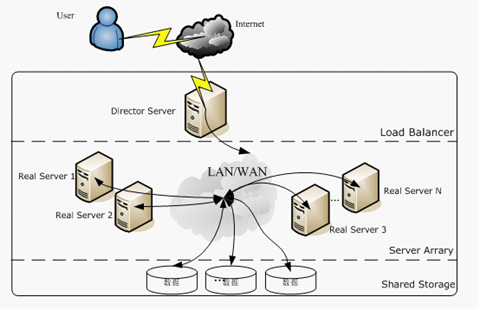时间序列预测之ARIMA

时间序列与平稳性

• 长期趋势(T)是在较长时期内受某种根本性因素作用而形成的总的变动趋势
• 季节变动(S)是在一年内随着季节的变化而发生的有规律的周期性变动。它是诸如气候条件、生产条件、节假日或人们的风俗习惯等各种因素影响的结果。
• 循环变动(C)是时间序列呈现出得非固定长度的周期性变动。循环波动的周期可能会持续一段时间，但与趋势不同，它不是朝着单一方向的持续变动，而是涨落相同的交替波动。
• 不规则变动(I)是时间序列中除去趋势、季节变动和周期波动之后的随机波动。不规则波动通常总是夹杂在时间序列中，致使时间序列产生一种波浪形或震荡式的变动。只含有随机波动的序列也称为平稳序列。

平稳性的判断标准

均值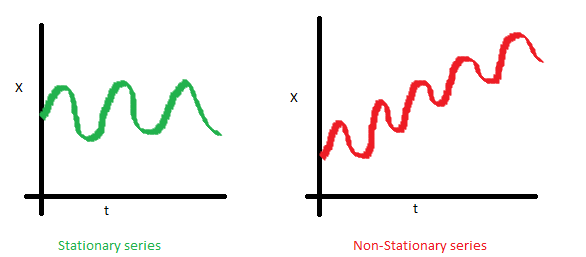方差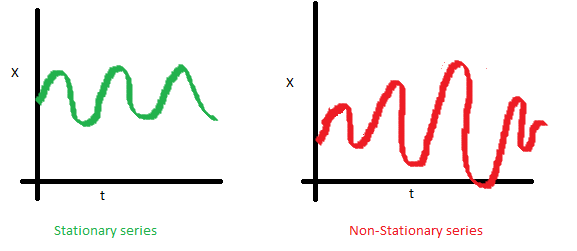协方差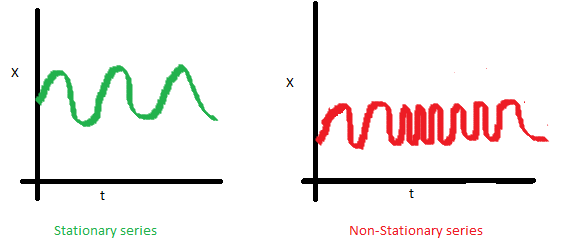平稳性检测方法

$$E(y_t) = E(y_{t+m})$$

$$cov(y_t, y_{t+k}) = cov(y_{t+k}, y_{t+k+m})$$

单位根检验

def test_stationarity(timeseries, window=12):
rolmean = timeseries.rolling(window=window, center=False).mean()
rolstd = timeseries.rolling(window=window, center=False).std()
orig = plt.plot(timeseries, color='blue', label='Original')# 设置原始图，移动平均图和标准差图的式样
mean = plt.plot(rolmean, color='red', label='Rolling Mean')
std = plt.plot(rolstd, color='black', label='Rolling Std')
plt.legend(loc='best')  # 使用自动最佳的图例显示位置
plt.title('Rolling Mean & Standard Deviation')
plt.show() #供肉眼观察是否平稳
dfoutput = pd.Series(dftest[0:4], index=['Test Statistic', 'p-value', 'Num Lags Used', 'Num Observations Used'])
for key, value in dftest.items():
dfoutput['Critical Value (%s)' % key] = value
print(dfoutput)
test_stationarity(ts_week)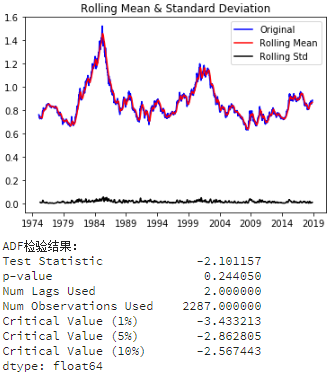• Test Statistic的值如果比Critical Value (5%)小则满足稳定性需求
• p-value越低（理论上需要低于05）证明序列越稳定。

白噪声检测

#生成一个伪随机白噪声用于测试acorr_ljungbox的可靠性
from statsmodels.stats.diagnostic import acorr_ljungbox  #导入白噪声检验函数
from random import gauss

whitenoise = pd.Series([gauss(0.0, 1.0) for i in range(1000)]) #创建一个高斯分布的白噪声
print(u'白噪声检验结果为：', acorr_ljungbox(whitenoise,lags=1)) #检验结果：平稳度，p-value。p-value>0.05为白噪声

时间序列转为平稳序列

• Deflation by CPI
• Logarithmic（取对数）
• First Difference（一阶差分）
• Seasonal Difference（季节差分）

• 趋势 Trend
• 季节性 Seasonality

加载数据

import pandas as pd
import numpy as np
import matplotlib.pylab as plt

ts = data['Passengers']

plt.plot(ts)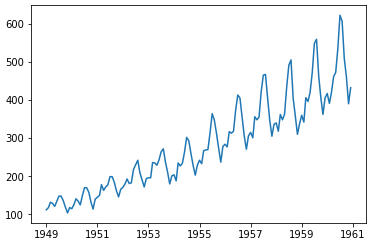检测稳定性

test_stationarity(ts)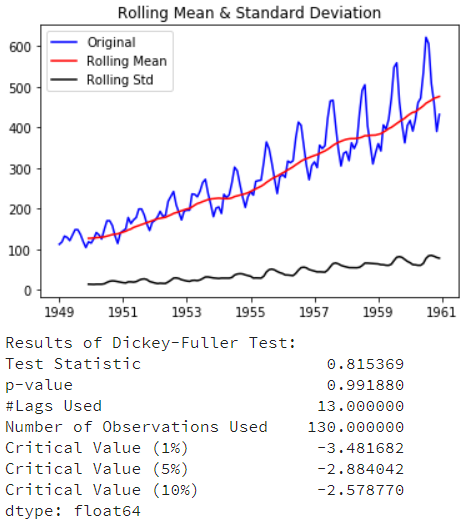消除趋势

• 将指数增长转为线性增长
• 可以平稳序列的方差
ts_log = np.log(ts)
plt.plot(ts_log)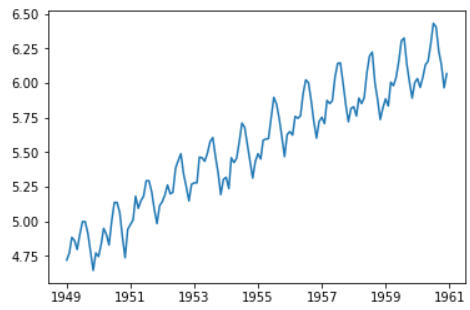• 聚合-取一段时间的平均值（月/周平均值）
• 平滑-取滚动平均数
• 多项式回归分析-适合的回归模型

moving_avg = ts_log.rolling(12).mean()
plt.plot(ts_log)
plt.plot(moving_avg, color='red')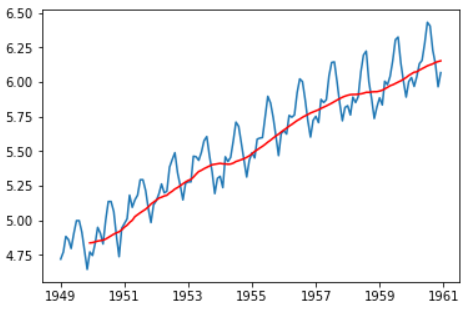ts_log_moving_avg_diff = ts_log - moving_avg
TravelDate
1949-01-01         NaN
1949-02-01         NaN
1949-03-01         NaN
1949-04-01         NaN
1949-05-01         NaN
1949-06-01         NaN
1949-07-01         NaN
1949-08-01         NaN
1949-09-01         NaN
1949-10-01         NaN
1949-11-01         NaN
1949-12-01   -0.065494
Name: Passengers, dtype: float64

ts_log_moving_avg_diff.dropna(inplace=True)
test_stationarity(ts_log_moving_avg_diff)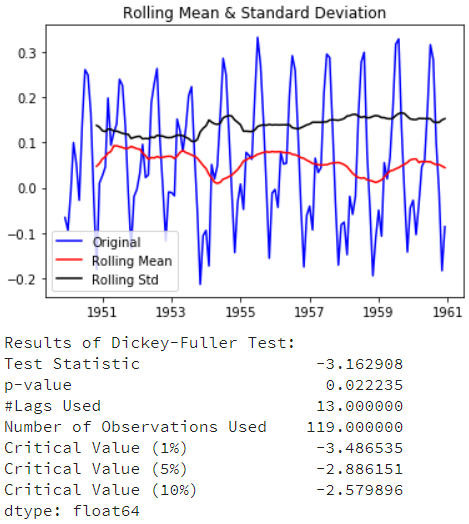expwighted_avg = ts_log.ewm(halflife=12).mean()
plt.plot(ts_log)
plt.plot(expwighted_avg, color='red')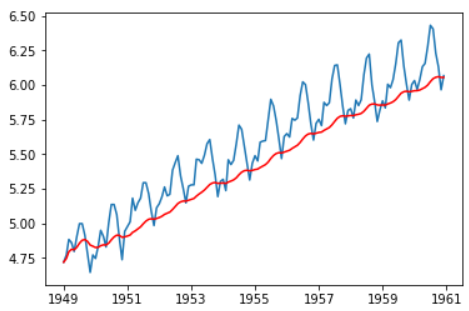ts_log_ewma_diff = ts_log - expwighted_avg
test_stationarity(ts_log_ewma_diff)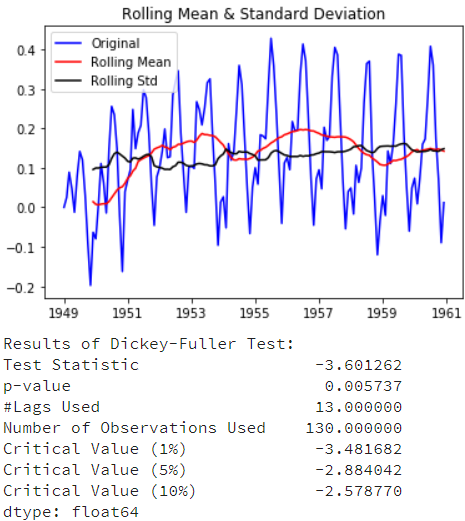消除季节性

• 差分：采用一个特定时间差的差值
• 分解：建立有关趋势和季节性的模型和从模型中删除它们

ts_log_diff = ts_log.diff() # ts_log_diff = ts_log - ts_log.shift()
plt.plot(ts_log_diff)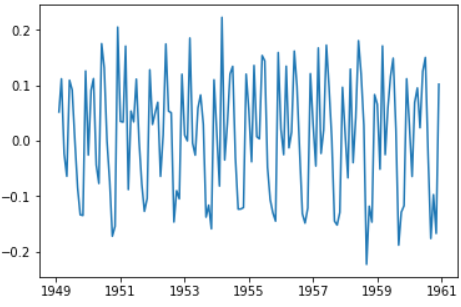ts_log_diff.dropna(inplace=True)
test_stationarity(ts_log_diff)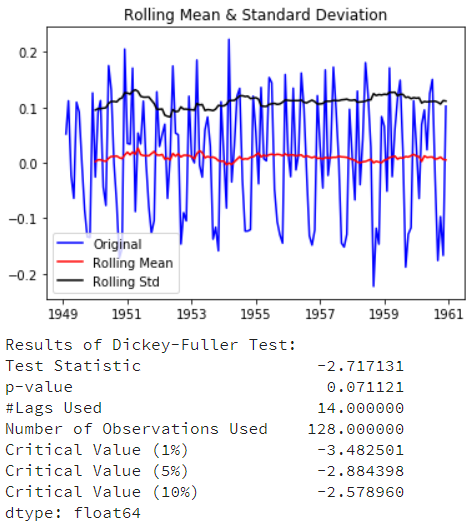statsmodels.tsa.seasonal.seasonal_decompose可以将时间序列的数据分解为趋势(trend),季节性(seasonality)和残差(residual)三部分：

from statsmodels.tsa.seasonal import seasonal_decompose
decomposition = seasonal_decompose(ts_log)

trend = decomposition.trend
seasonal = decomposition.seasonal
residual = decomposition.resid

plt.subplot(411)
plt.plot(ts_log, label='Original')
plt.legend(loc='best')
plt.subplot(412)
plt.plot(trend, label='Trend')
plt.legend(loc='best')
plt.subplot(413)
plt.plot(seasonal,label='Seasonality')
plt.legend(loc='best')
plt.subplot(414)
plt.plot(residual, label='Residuals')
plt.legend(loc='best')
plt.tight_layout()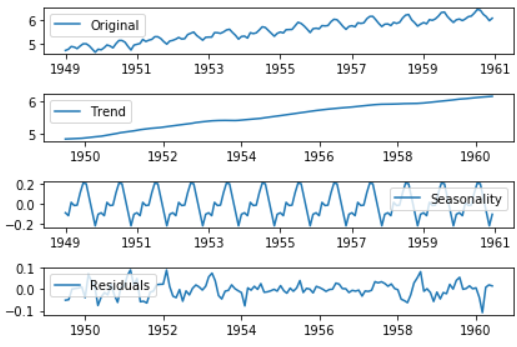ts_log_decompose = residual
ts_log_decompose.dropna(inplace=True)
test_stationarity(ts_log_decompose)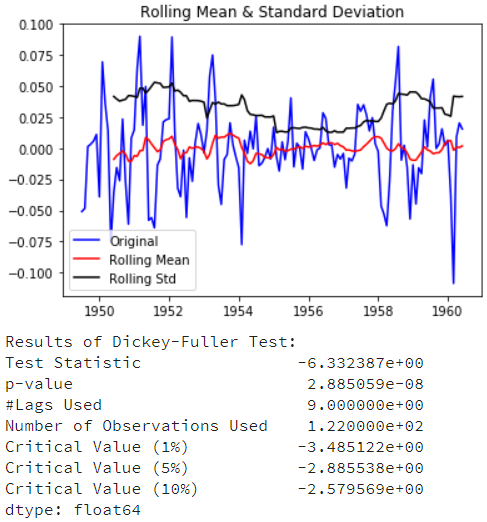DF测试统计量明显低于1%的临界值，这样时间序列是非常接近稳定。

时间序列建模

相关系数与自相关函数

相关系数

$$\cos<\vec{a},\vec{b}>=\frac{\vec{a}\cdot \vec{b}}{|\vec{a}||\vec{b}|}$$

$$(x_1,y_1)\cdot (x_2,y_2)={x_1}{x_2}+{y_1}{y_2}$$

$$\rho_{xy} = \frac{Cov(X,Y)}{\sqrt{Var(X)Var(Y)}}$$

$$\rho_{xy} = \frac{\sum_{t=1}^{T}(x_t- \bar x)(y_t-\bar y)}{\sqrt{ \sum_{t=1}^{T}(x_t- \bar x)^{2}\sum_{t=1}^{T}(y_t- \bar y)^{2}}} = \frac{\overrightarrow{(X- \bar x)} \cdot \overrightarrow{(Y- \bar y)}}{| \overrightarrow{(X- \bar x)} || \overrightarrow{(Y- \bar y)} |}$$

自相关函数(Autocorrelation Function, ACF)

$r_t$与$r_{t-l}$的相关系数称为$r_t$的间隔为$l$的自相关系数，通常记为$\rho_l$：

$$\rho_l = \frac{Cov(r_t,r_{t-l})} {\sqrt{Var(r_t)Var(r_{t-l})}} = \frac{Cov(r_t,r_{t-l})} {Var(r_t)}$$

$$\hat \rho_l = \frac{\sum_{t=l+1}^{T}(r_t- \bar r)(r_{t-l}-\bar r)}{ \sum_{t=1}^{T}(r_t- \bar r)^{2}}, 0 \leq l \leq T-1$$

$$H0: \rho_1 = … = \rho_m=0$$

$$H1: \exists i \in \{1,…,m\}, \rho_i \ne 0$$

$$Q(m) = T(T+2) \sum_{l=1}^{m} \frac{\hat{\rho_l}^{2}}{T-l}$$

$Q(m)$渐进服从自由度为$m$的$\chi^2$分布

$$Q(m) > \chi_\alpha^{2} ,拒绝H_0$$

import pandas as pd
import numpy as np
import matplotlib.pyplot as plt

import tushare as ts
pro = ts.pro_api()
df = pro.daily(ts_code='600519.SH',start_date='20180101') #茅台股价

data['change_pct'] =''
for i in range(0,len(data['close'])-1):
data.iloc[i, 1] = 100*(data['close'][i+1] - data['close'][i])/data['close'][i] # 计算每日收益率（涨跌幅）
data['change_pct'] = data['change_pct'][0:-1]
data['close_diff_1'] = data['close'].diff(1)
data = data[0:-1] # 由于changepct值最后一行缺失，因此去除最后一行
data['change_pct'] = data['change_pct'].astype('float64') #使changepct数据类型和其他三列保持一致

data.plot(subplots=True,figsize=(12,12))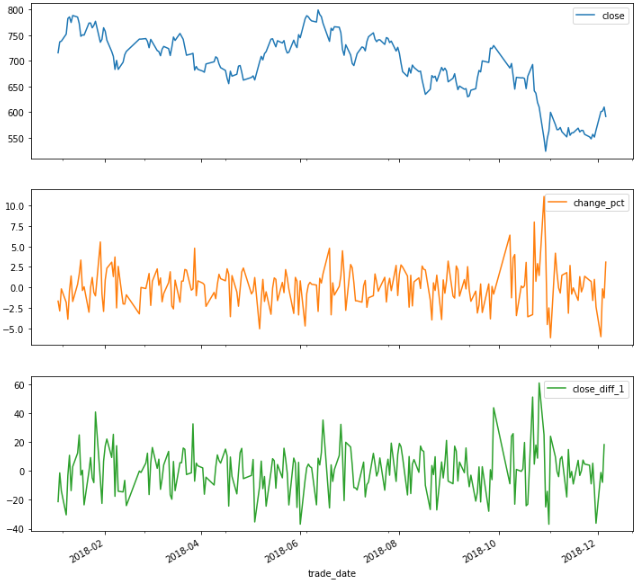import statsmodels.api as sm
close_data = data['close'] # 上证指数每日收盘价
m = 10 # 检验10个自相关系数

acf,q,p = sm.tsa.acf(close_data, nlags=m, qstat = True)

# np.r_是按列连接两个矩阵，就是把两矩阵上下相加，要求列数相等，类似于pandas中的concat()。
# np.c_是按行连接两个矩阵，就是把两矩阵左右相加，要求行数相等，类似于pandas中的merge()。
out = np.c_[range(1,11), acf[1:], q, p]

output = pd.DataFrame(out, columns=['lag', 'ACF', 'Q', 'P-value'])
output = output.set_index('lag')
print(output)
lag        ACF            Q        P-value
1.0   0.963283   212.504727   3.903196e-48
2.0   0.928599   410.863584   6.054892e-90
3.0   0.893812   595.463129  9.703299e-129
4.0   0.858595   766.569712  1.337073e-164
5.0   0.820359   923.482644  2.201222e-197
6.0   0.778788  1065.538402  5.955998e-227
7.0   0.744518  1195.960070  5.278705e-254
8.0   0.712521  1315.960270  8.343090e-279
9.0   0.686764  1427.955165  7.044640e-302
10.0  0.658661  1531.448754   0.000000e+00

change_data = data['change_pct']
m = 10 # 检验10个自相关系数

acf,q,p = sm.tsa.acf(change_data,nlags=m,qstat=True)  ## 计算自相关系数 及p-value
out = np.c_[range(1,11), acf[1:], q, p]
output=pd.DataFrame(out, columns=['lag', "AC", "Q", "P-value"])
output = output.set_index('lag')
print(output)
lag         AC         Q   P-value
1.0   0.010467  0.025092  0.874137
2.0  -0.018733  0.105814  0.948468
3.0  -0.015576  0.161875  0.983496
4.0  -0.023547  0.290575  0.990414
5.0   0.034672  0.570871  0.989298
6.0  -0.133740  4.760213  0.574915
7.0  -0.047866  5.299284  0.623491
8.0  -0.085952  7.045520  0.531729
9.0   0.064843  8.043923  0.529726
10.0 -0.032424  8.294713  0.600074

自相关图与偏相关图详解

import pandas as pd
from matplotlib import pyplot as plt
data.plot(figsize=(12,6))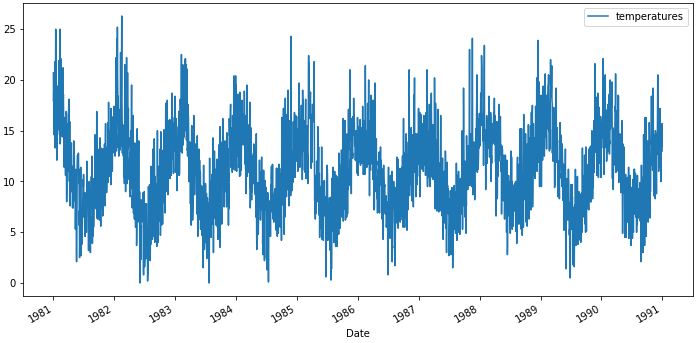相关与自相关

import statsmodels.api as sm
sm.graphics.tsa.plot_acf(data)
plt.figure(figsize=(12, 6))
plt.show()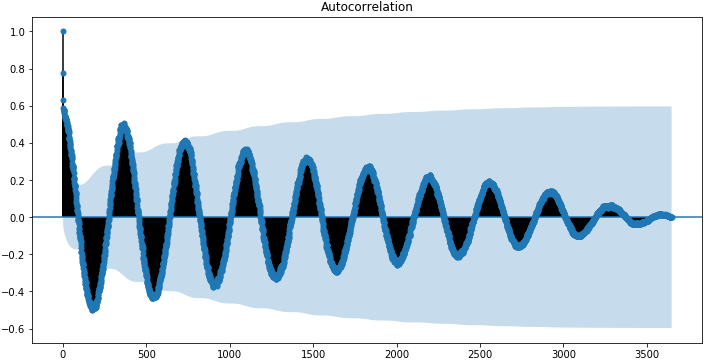sm.graphics.tsa.plot_acf(data, lags=50)
plt.figure(figsize=(12, 6))
plt.show()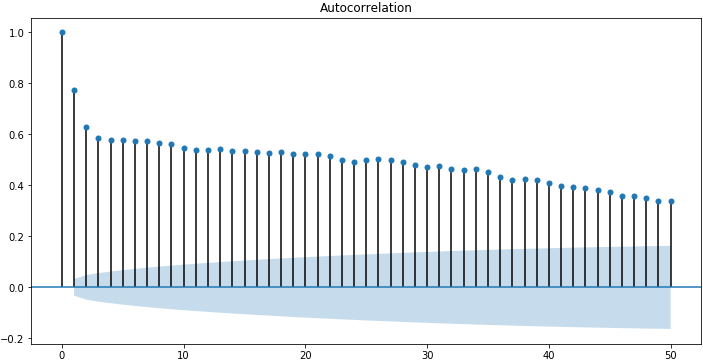偏自相关函数（PACF）

sm.graphics.tsa.plot_pacf(data, lags=50)
plt.figure(figsize=(12, 6))
plt.show()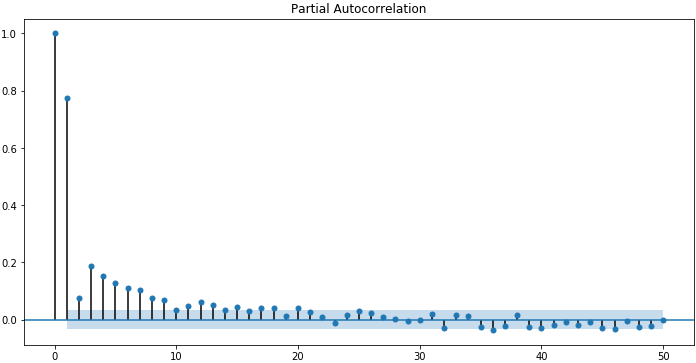ACF和PACF图的直观认识

• 自相关函数 (ACF)。延迟为 k 时，这是相距 k 个时间间隔的序列值之间的相关性。
• 偏自相关函数 (PACF)。延迟为 k 时，这是相距 k 个时间间隔的序列值之间的相关性，同时考虑了间隔之间的值。

截尾与拖尾是什么鬼？

• 截尾：在大于某个常数k后快速趋于0为k阶截尾
• 拖尾：始终有非零取值，不会在k大于某个常数后就恒等于零(或在0附近随机波动)

$$z_t=\varphi _{1}z_{t-1}+\varphi _{2}z_{t-2}+…+\varphi _{p}z_{t-p}+\alpha _{t}-\theta _{1}\alpha _{t-1}-…-\theta _{p}\alpha _{t-p}$$

$$\varphi _{p}(B)z_{t} = \theta _{q}(B)\alpha _(t)$$

$$\varphi _{p}(B) = 1 – \varphi _{1}B – \varphi _{2}B^2 – … – \varphi _{p}B^p$$

$$\theta _{q}(B) = 1 – \theta _{1}B – \theta _{2}B^2 – … – \theta _{q}B^q$$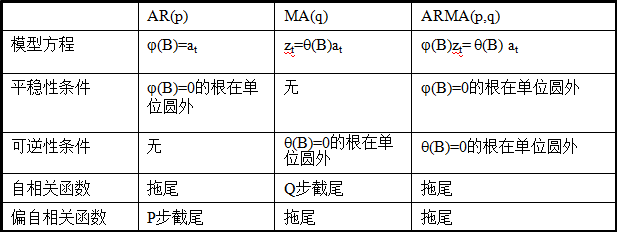白噪声序列与线性时间序列

线性时间序列

$$r_t = \mu + \sum_{i=0}^{\infty}\psi_ia_{t-i}$$

$\mu$为$r_t$的均值， $psi_0=1,\{a_t\}$为白噪声序列，则我们称$\{r_t\}$为线性序列。其中$a_t$称为在$t$时刻的新息(innovation)扰动(shock)

$$E(r_t) = \mu , Var(r_t) = \sigma_a^2 \sum_{i=0}^{\infty} \psi_i^{2}$$

$\sigma_a^2$为$a_t$的方差。因为$Var(r_t)$一定小于正无穷，因此$\{\psi_i^2\}$必须是收敛序列，因此满足 $i \to \infty$ 时， $\psi_i^2 \to 0$。即，随着$i$的增大，远处的扰动$a_{t-i}$对$r_t$的影响会逐渐消失。

自回归模型(Auto Regressive, AR)

AR 模型的理解

$$x(t) = \alpha * x(t–1) + error(t)$$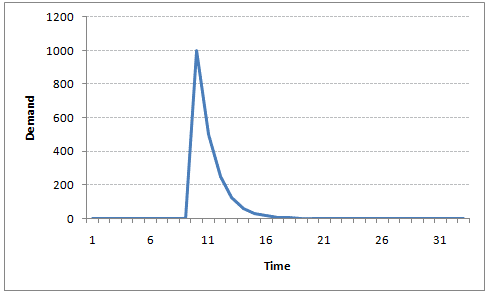$$r_t = \phi_0 + \phi_1r_{t-1} + a_t$$

$$r_t = \phi_0 + \phi_1r_{t-1} + \cdots + \phi_pr_{t-p}+ a_t \qquad (2.0)$$

AR(p)模型的特征根及平稳性检验

$$E(r_t) = \mu \qquad Var(r_t) = \gamma_0 \qquad Cov(r_t,r_{t-j})=\gamma_j$$

$$E(a_t) = 0, Var(a_t) = \sigma_a^2$$

$$E(r_t) = \phi_0 + \phi_1E(r_{t-1}) + \phi_2E(r_{t-2}) + \cdots + \phi_pE(r_{t-p})$$

$$\mu = \phi_0 + \phi_1\mu + \phi_2\mu+ \cdots +\phi_p\mu\\$$

$$E(r_t)=\mu=\frac{\phi_0}{1-\phi_1 – \phi_2 – \cdots -\phi_p} \qquad$$

$$1 – \phi_1x – \phi_2x^2 – \cdots -\phi_px^p = 0$$

change_data = data['change_pct'] # 载入收益率序列
change_data = np.array(change_data, dtype=np.float)
model = sm.tsa.AR(change_data)
results_AR = model.fit()
plt.figure(figsize=(12,6))
plt.plot(change_data, 'b', label = 'changepct')
plt.plot(results_AR.fittedvalues, 'r', label = 'AR model')
plt.legend()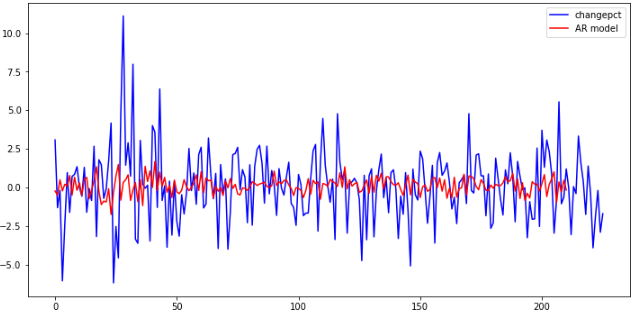print(len(results_AR.roots)) # 模型阶数

r1 = 1
theta = np.linspace(0, 2*np.pi, 360)
x1 = r1 * np.cos(theta)
y1 = r1 * np.sin(theta)
plt.figure(figsize=(6,6))
plt.plot(x1, y1, 'k')  # 画单位圆
roots = 1 / results_AR.roots  # 计算results_AR.roots后取倒数
for i in range(len(roots)):
plt.plot(roots[i].real, roots[i].imag, '.r', markersize=8)  #画特征根
plt.show()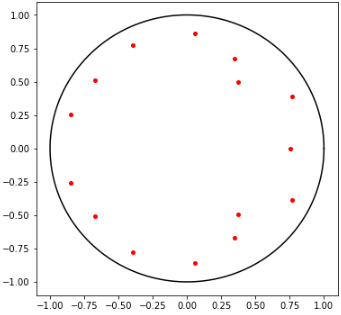AR模型的阶数确定

$$r_t = \phi_{0,1} + \phi_{1,1}r_{t-1} + \epsilon_{1t}$$

$$r_t = \phi_{0,2} + \phi_{1,2}r_{t-1} + \phi_{2,2}r_{t-2} + \epsilon_{2t}$$

$$r_t = \phi_{0,3} + \phi_{1,3}r_{t-1} + \phi_{2,3}r_{t-2} + \phi_{3,3}r_{t-3} + \epsilon_{3t}$$

$$r_t = \phi_{0,4} + \phi_{1,4}r_{t-1} + \phi_{2,4}r_{t-2} + \phi_{3,4}r_{t-3} + \phi_{4,4}r_{t-4} + \epsilon_{4t}$$

$$\dots \dots \dots \dots$$

$$\frac{2}{\sqrt{n}} = \frac{2}{\sqrt{243}}$$

fig = plt.figure(figsize=(12,6))
fig = sm.graphics.tsa.plot_pacf(change_data, lags=40, ax=ax1)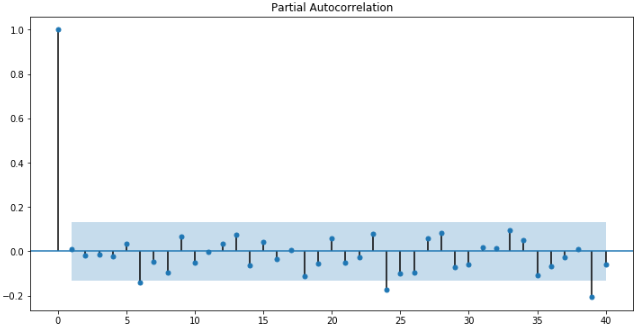信息准则函数

$$AIC = -2 \ln L + 2 k$$

$$BIC = -2 \ln L + \ln n \cdot k$$

$$HQIC = -2 \ln L + \ln(\ln n) \cdot k$$

aicList = []
bicList = []
hqicList = []
for i in range(1,8):  #从1阶开始算
order = (i,0)  # 使用ARMA模型，其中MA阶数为0，只考虑了AR阶数。
chgdataModel = sm.tsa.ARMA(change_data,order).fit()
aicList.append(chgdataModel.aic)
bicList.append(chgdataModel.bic)
hqicList.append(chgdataModel.hqic)

plt.figure(figsize=(10,6))
plt.plot(aicList,'ro--',label='aic value')
plt.plot(bicList,'bo--',label='bic value')
plt.plot(hqicList,'ko--',label='hqic value')
plt.legend(loc=0)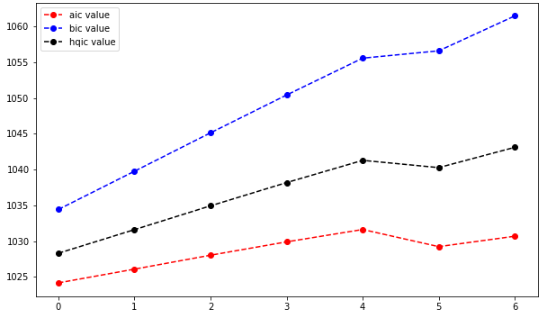AR模型的检验

delta = results_AR.fittedvalues - change_data[15:] # 取得误差项
plt.figure(figsize=(10,6))
# plt.plot(change_data[17:],label='original value')
# plt.plot(results_AR.fittedvalues,label='fitted value')
plt.plot(delta,'r',label=' residual error')
plt.legend(loc=0)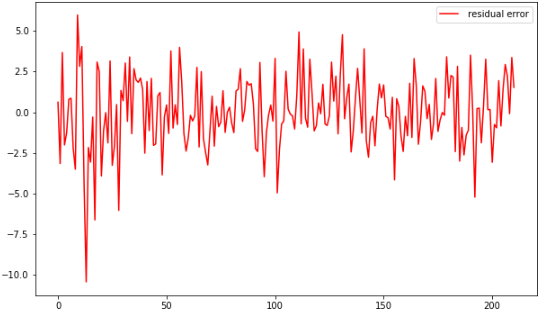acf,q,p = sm.tsa.acf(delta,nlags=10,qstat=True)  # 计算自相关系数 及p-value
out = np.c_[range(1,11), acf[1:], q, p]
output=pd.DataFrame(out, columns=['lag', "ACF", "Q", "P-value"])
output = output.set_index('lag')
print(output)
lag        ACF         Q   P-value
1.0   0.001303  0.000363  0.984792
2.0  -0.003122  0.002459  0.998771
3.0   0.013582  0.042318  0.997714
4.0  -0.014884  0.090414  0.999008
5.0   0.014418  0.135770  0.999656
6.0   0.007551  0.148269  0.999936
7.0  -0.003774  0.151407  0.999990
8.0  -0.014438  0.197556  0.999996
9.0   0.022536  0.310547  0.999996
10.0 -0.014879  0.360051  0.999999

拟合优度

$$AdjR^2 = 1 – \frac{\hat{\sigma}^2_{\epsilon}}{\hat{\sigma}^2_{r_t}}$$

AR模型的预测

train = change_data[:-10]
test = change_data[-10:]
output = sm.tsa.AR(train).fit()
output.predict()

predicts = output.predict(216, 225, dynamic=True) #共226个数据
compare = pd.DataFrame()
compare['original'] = change_data[-10:]
compare['predict'] = predicts
print(compare)
original   predict
0  1.608146  0.853237
1  0.388352 -0.585180
2 -1.726237  0.435650
3  1.406797  0.482424
4 -0.406002  0.967699
5 -3.883607 -0.727744
6 -1.830801  0.412699
7 -0.174712 -0.076781
8 -2.877610  0.223773
9 -1.677702 -0.055838
compare.plot()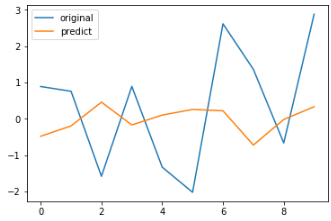滑动平均模型（Moving Average, MA）

MA模型的理解

$$x(t) = beta * error(t-1) + error (t)$$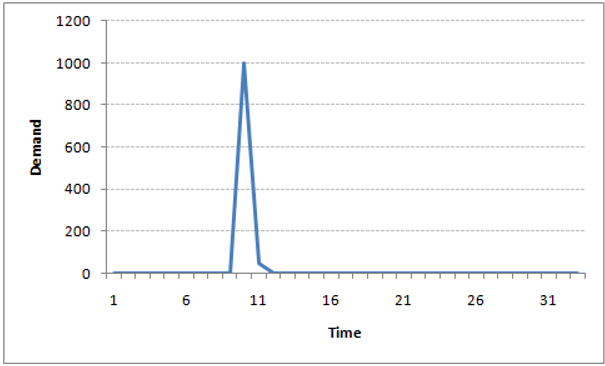$$r_t = c_0 + a_t – \theta_1a_{t-1} – \cdots – \theta_qa_{t-q}$$

$c_0$为一个常数项。这里的$a_t$,是AR模型$t$时刻的扰动或者说新息，则可以发现，该模型，使用了过去q个时期的随机干扰或预测误差来线性表达当前的预测值。

MA模型的性质

MA模型总是弱平稳的，因为他们是白噪声序列（残差序列）的有限线性组合。因此，根据弱平稳的性质可以得出两个结论：

$$E(r_t) = c_0$$

$$Var(r_t) = (1+ \theta_1^2 + \theta_2^2 + \cdots +\theta_q^2)\sigma_a^2$$

1阶：$|\theta_1| < 1$

2阶：$|\theta_2| < 1, \theta_1 + \theta_2 < 1$

MA模型的的阶数确定

fig2 = plt.figure(figsize=(12,6))
fig2 = sm.graphics.tsa.plot_acf(change_data,lags=40,ax=ax2)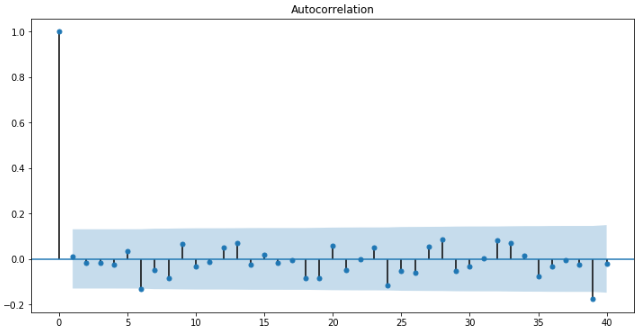results_MA = sm.tsa.ARMA(change_data, (0,6)).fit() # 取ARMA模型中AR的阶数为0，MA的阶数为6
plt.figure(figsize=(12,6))
plt.plot(change_data, 'b', label = 'changepct')
plt.plot(results_MA.fittedvalues, 'r', label = 'MA model')
plt.legend()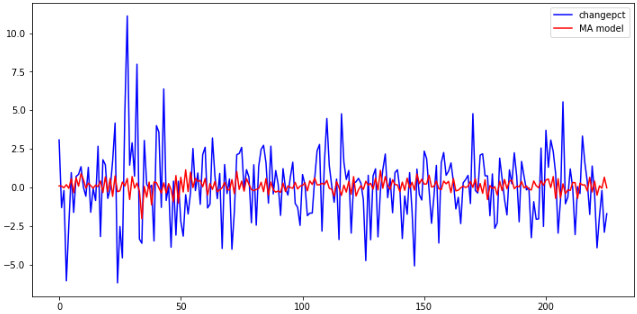MA模型的预测

train = change_data[:-10]
test = change_data[-10:]
chgdataModel_MA = sm.tsa.ARMA(train, (0,6)).fit() # 取ARMA模型中AR的阶数为0，MA的阶数为6

delta = chgdataModel_MA.fittedvalues - train
score = 1 - delta.var()/train.var()
print(score)

predicts_MA = chgdataModel_MA.predict(216, 225, dynamic=True)
compare_MA = pd.DataFrame()
compare_MA['original'] = test
compare_MA['predict_MA'] = predicts_MA
print(compare_MA)

自回归-滑动平均模型（ARMA）

AR模型与MA模型的不同

AR和MA 模型之间的主要区别是基于不同时间点的时间序列对象之间的相关性。x(t)与x(t-n)的相关性总为0，这直接源于 x(t)和 x(t-n)之间的协方差对于MA模型来说是零。然而，AM模型中x(t)与x(t-1)的相关性随着时间的推移变得越来越小。不管是否有 AR 模型或 MA 模型，这种差异都可以被利用。

$$r_t = \phi_0 + \sum_{i=1}^p\phi_ir_{t-i} + a_t + \sum_{i=1}^q\theta_ia_{t-i}$$

$$(1-\phi_1B – \cdots -\phi_pB^p)r_t = \phi_0 + (1-\theta_1B-\cdots – \theta_qB^q)a_t$$

$$E(r_t) = \frac{\phi_0}{1-\phi_1-\cdots – \phi_p}$$

$$1 – \phi_1x – \phi_2x^2 – \cdots -\phi_px^p = 0$$

ACF是不同滞后函数之间的总关联图。例如，在GDP问题上，时间点T的GDP是x(t)。我们对x(t)与x(t-1)、x(t-2)等的相关性很感兴趣。现在让我们来反思一下上面所学的内容。在移动平均序列的滞后n中，x(t)与x(t-n-1)之间不存在任何相关。因此，总相关图在第n 次滞后处截止。因此，很容易找到一个MA系列的滞后。对于AR 序列，这种相关性将逐渐下降而没有任何截止值。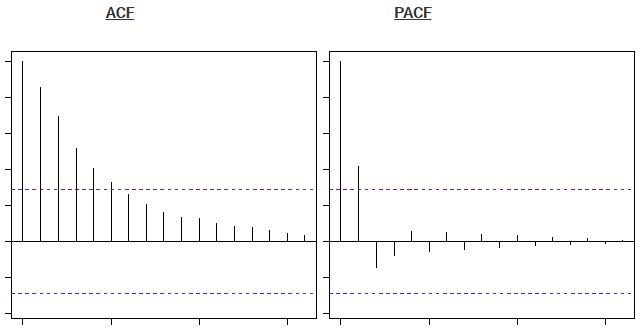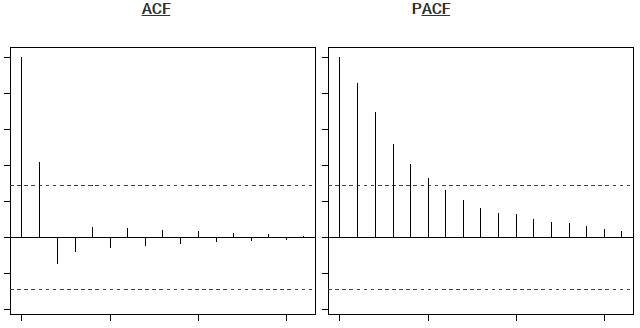ARMA模型的阶数确定

PACF、ACF 判断模型阶数

fig = plt.figure(figsize=(12,12))
fig = sm.graphics.tsa.plot_acf(change_data,lags=30,ax=ax1)
fig = sm.graphics.tsa.plot_pacf(change_data,lags=30,ax=ax2)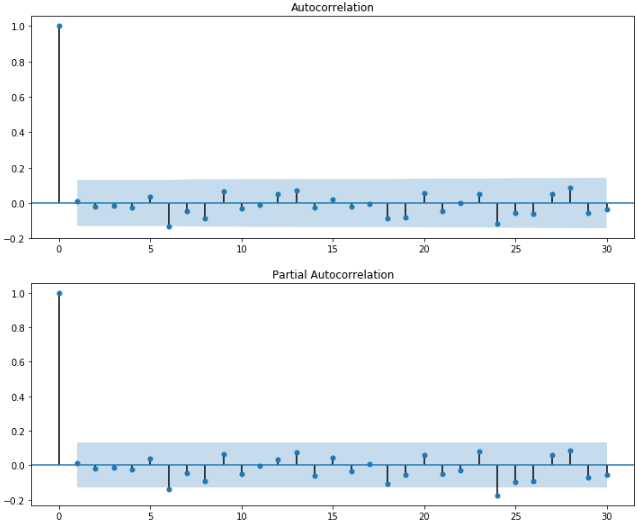import warnings
warnings.filterwarnings("ignore")
sm.tsa.arma_order_select_ic(change_data,max_ar=6,max_ma=4,ic='aic')['aic_min_order']  # AIC
#(5, 4)

sm.tsa.arma_order_select_ic(change_data,max_ar=6,max_ma=4,ic='bic')['bic_min_order']  # BIC
#(0, 0)

sm.tsa.arma_order_select_ic(change_data,max_ar=6,max_ma=4,ic='hqic')['hqic_min_order'] # HQIC
#(0, 0)

ARMA模型的预测和检验

• 信息准则定阶时，为减少计算量，我们设定了AR和MA模型的最大阶数，这样得到的阶数只是局部最优解。
• ARMA模型不足以预测股价的变化趋势（这也正常，不然人人都可以赚大钱了）

差分自回归滑动平均模型（Auto-Regressive Integrated Moving Averages，ARIMA）

• Number of AR (Auto-Regressive) terms (p)： 现在点使用多少个过往数据计算。AR条件仅仅是因变量的滞后。如：如果P等于5，那么预测x（t）将是x（t-1）…x（t-5）。
• Number of MA (Moving Average) terms (q)：使用多少个过往的残余错误值。MA条件是预测方程的滞后预测错误。如：如果q等于5，预测x（t）将是e（t-1）…e(t-5)，e(i)是移动平均叔在第i个瞬间和实际值的差值。
• Number of Differences (d)：为时间序列成为平稳时所做的差分次数。有非季节性的差值，这种情况下我们采用一阶差分。

ARIMA模型分析框架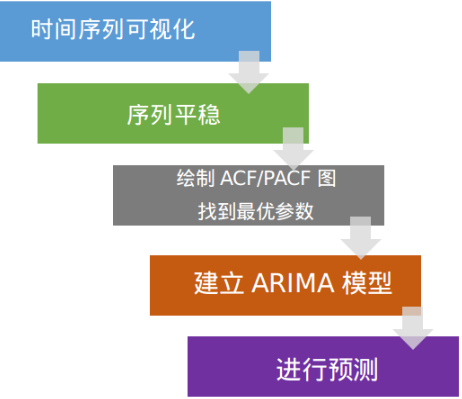1、消除趋势：这里我们简单的删除时间序列中的趋势成分。例如，我的时间序列的方程是：

$$x(t) = (mean + trend * t) + error$$

2、差分：这是常用的去除非平稳性的技术。这里我们是对序列的差分的结果建立模型而不是真正的序列。例如：

$$x(t) – x(t-1) = ARMA (p, q)$$

• p:AR
• d:I
• q:MA

3、季节性：季节性直接被纳入ARIMA模型中。下面的应用部分我们再讨论这个。

ARIMA模型参数p、d、q

import pandas as pd
import matplotlib.pyplot as plt
import statsmodels.api as sm

data.plot(figsize=(12,6))ARIMA 模型对时间序列的要求是平稳型。因此，当你得到一个非平稳的时间序列时，首先要做的即是做时间序列的差分，直到得到一个平稳时间序列。如果你对时间序列做d次差分才能得到一个平稳序列，那么可以使用ARIMA(p,d,q)模型，其中d是差分次数。

1阶差分：

diff1 = data.diff(1)
diff1.plot(figsize=(12,6))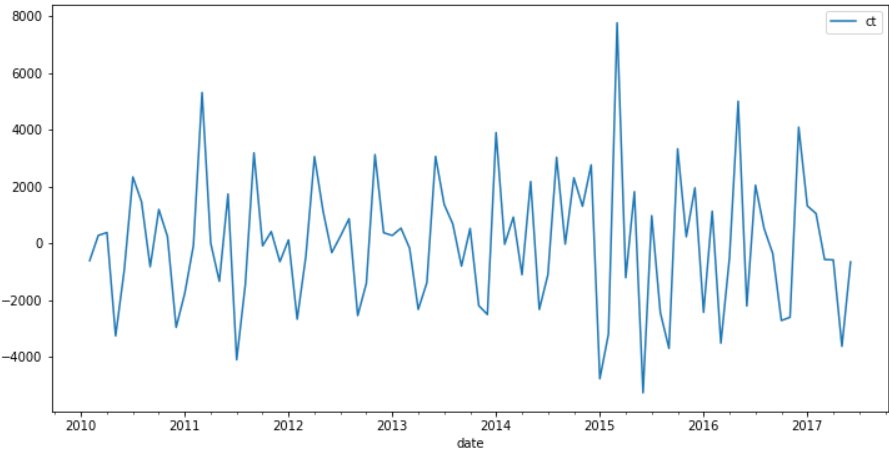diff2 = data.diff(2)
diff2.plot(figsize=(12,6))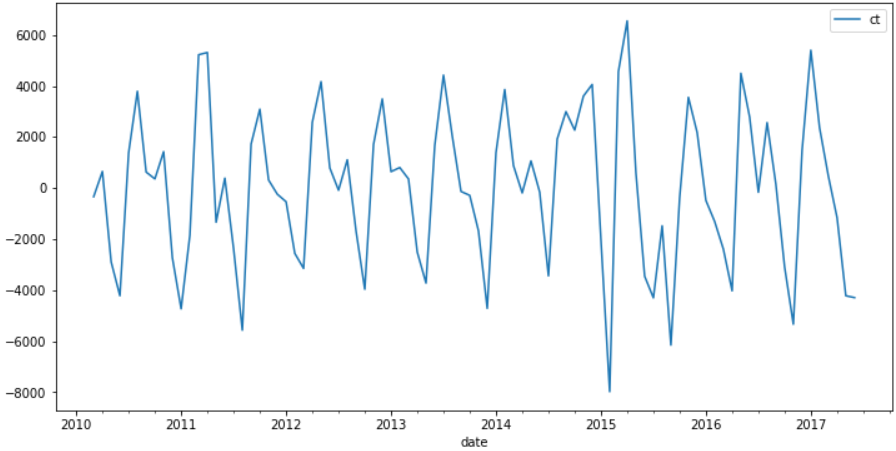• 假如时间序列在很高的lag（10以上）上有正的ACF，则需要很高阶的差分
• 假如lag-1的ACF是0或负数或者非常小，则不需要很高的差分，假如lag-1的ACF是-0.5或更小，序列可能overdifferenced。
• 最优的差分阶数一般在最优阶数下标准差最小（但并不是总数如此）
• 模型不查分意味着原先序列是平稳的；1阶差分意味着原先序列有个固定的平均趋势；二阶差分意味着原先序列有个随时间变化的趋势
• 模型没有差分一般都有常数项；有两阶差分一般没常数项；假如1阶差分模型非零的平均趋势，则有常数项

• 假如PACF显示截尾或者lag-1的ACF是正的（此时序列仍然有点underdifferenced），则需要考虑AR项；PACF的截尾项表明AR的阶数
• 假如ACF显示截尾或者lag-1的ACF是负的（此时序列有点overdifferenced），则需要加MA项，ACF的截尾项表明AR的阶数
• AR和MA可以相互抵消对方的影响，所以假如用AR-MA模型去拟合数据，仍然需要考虑加些AR或MA项。尤其在原先模型需要超过10次的迭代去converge。
• 假如在AR部分有个单位根（AR系数和大约为1），此时应该减少一项AR增加一次差分
• 假如在MA部分有个单位根（MA系数和大约为1），此时应该减少一项AR减少一次差分
• 假如长期预测出现不稳定，则可能AR、MA系数有单位根

diff1.dropna(inplace=True)
fig = plt.figure(figsize=(12,8))
fig = sm.graphics.tsa.plot_acf(diff1,lags=40,ax=ax1)
fig = sm.graphics.tsa.plot_pacf(diff1,lags=40,ax=ax2)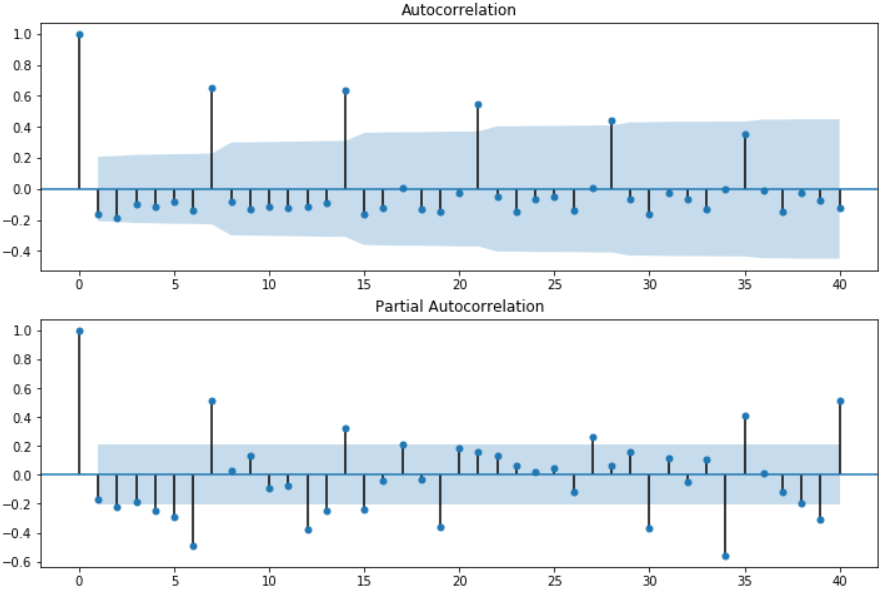• 自相关图显示滞后有3（4）个阶超出了置信边界
• 偏相关图显示在滞后1至7阶（lags 1,2,…，7）时的偏自相关系数超出了置信边界，从lag 7之后偏自相关系数值缩小至0

• ARMA(0,1)模型：即自相关图在滞后1阶之后缩小为0，且偏自相关缩小至0，则是一个阶数q=1的移动平均模型；
• ARMA(7,0)模型：即偏自相关图在滞后7阶之后缩小为0，且自相关缩小至0，则是一个阶层p=3的自回归模型；
• ARMA(7,1)模型：即使得自相关和偏自相关都缩小至零。则是一个混合模型。

arma_mod70 = sm.tsa.ARMA(diff1,(7,0)).fit()
print("arma_mod70:",arma_mod70.aic,arma_mod70.bic,arma_mod70.hqic)
arma_mod01 = sm.tsa.ARMA(diff1,(0,1)).fit()
print("arma_mod01:",arma_mod01.aic,arma_mod01.bic,arma_mod01.hqic)
arma_mod71 = sm.tsa.ARMA(diff1,(7,1)).fit()
print("arma_mod71:",arma_mod71.aic,arma_mod71.bic,arma_mod71.hqic)

arma_mod70: 1579.7025547690232 1602.1002820966125 1588.7304359010805
arma_mod01: 1632.3203732818517 1639.7862823910482 1635.3296669925376
arma_mod71: 1581.0916055163707 1605.9779692136922 1591.12258455199

ARIMA模型的校验

resid = arma_mod70.resid#残差
fig = plt.figure(figsize=(12,8))
fig = sm.graphics.tsa.plot_acf(resid.values.squeeze(), lags=40, ax=ax1)
fig = sm.graphics.tsa.plot_pacf(resid, lags=40, ax=ax2)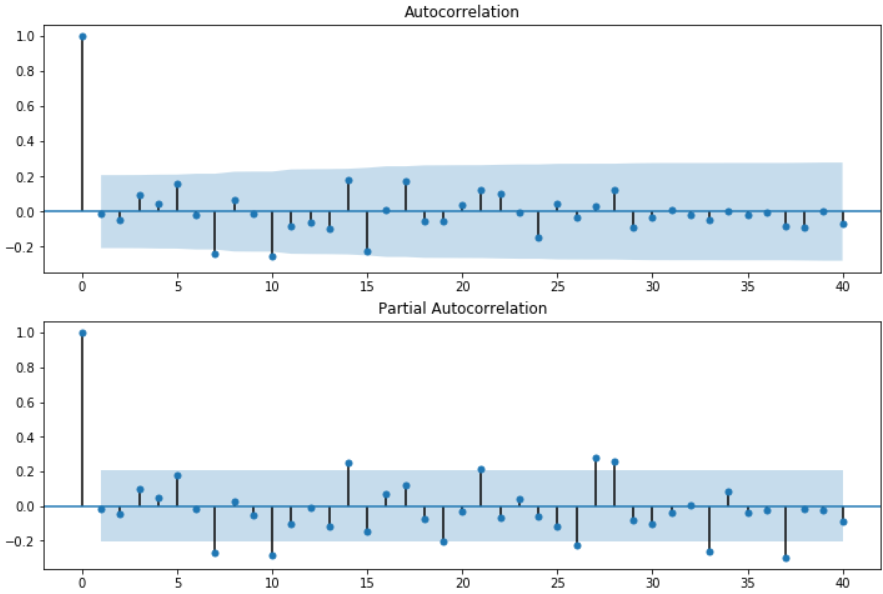D－W检验

Durbin-Watson检验，简称D-W检验，是目前检验自相关性最常用的方法，但它只使用于检验一阶自相关性。当DW值显著的接近于O或４时，则存在自相关性，而接近于２时，则不存在（一阶）自相关性。

print(sm.stats.durbin_watson(resid))
# 2.024244082702278

from statsmodels.graphics.api import qqplot
fig = plt.figure(figsize=(12,6))
fig = qqplot(resid, line='q', ax=ax, fit=True)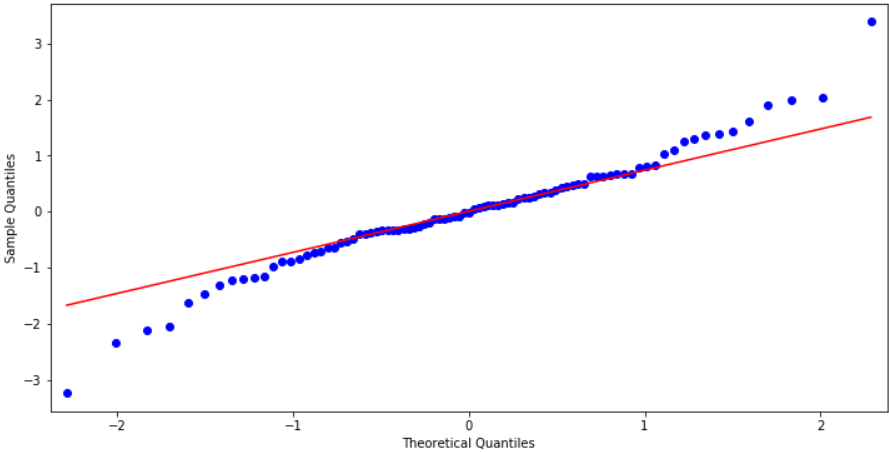Ljung-Box检验

Ljung-Box test是对randomness的检验,或者说是对时间序列是否存在滞后相关的一种统计检验。对于滞后相关的检验，我们常常采用的方法还包括计算ACF和PCAF并观察其图像，但是无论是ACF还是PACF都仅仅考虑是否存在某一特定滞后阶数的相关。LB检验则是基于一系列滞后阶数，判断序列总体的相关性或者说随机性是否存在。 时间序列中一个最基本的模型就是高斯白噪声序列。而对于ARIMA模型，其残差被假定为高斯白噪声序列，所以当我们用ARIMA模型去拟合数据时，拟合后我们要对残差的估计序列进行LB检验，判断其是否是高斯白噪声，如果不是，那么就说明ARIMA模型也许并不是一个适合样本的模型。

import numpy as np

r,q,p = sm.tsa.acf(resid.values.squeeze(), qstat=True)
data = np.c_[range(1,41), r[1:], q, p]
table = pd.DataFrame(data, columns=['lag', "AC", "Q", "Prob(>Q)"])
print(table.set_index('lag'))
AC          Q  Prob(>Q)
lag
1.0  -0.014445   0.019203  0.889787
2.0  -0.047441   0.228719  0.891937
3.0   0.097778   1.129072  0.770061
4.0   0.047514   1.344178  0.853837
5.0   0.156219   3.697174  0.593785
6.0  -0.017855   3.728282  0.713391
7.0  -0.241230   9.475812  0.220274
8.0   0.068078   9.939217  0.269318
9.0  -0.012041   9.953895  0.354231
10.0 -0.256684  16.708543  0.081067
11.0 -0.085178  17.461885  0.094936
12.0 -0.063577  17.887029  0.119164
13.0 -0.096512  18.879634  0.126883
14.0  0.181119  22.422039  0.070348
15.0 -0.223097  27.869400  0.022401
16.0  0.012916  27.887910  0.032608
17.0  0.176769  31.402779  0.017833
18.0 -0.053140  31.724897  0.023694
19.0 -0.057704  32.110150  0.030374
20.0  0.037425  32.274556  0.040459
21.0  0.120519  34.004510  0.036199
22.0  0.102662  35.278536  0.036225
23.0 -0.007830  35.286058  0.048710
24.0 -0.148547  38.035517  0.034383
25.0  0.046254  38.306261  0.043173
26.0 -0.032621  38.443060  0.055055
27.0  0.032381  38.580025  0.069141
28.0  0.124968  40.653492  0.057760
29.0 -0.092711  41.813707  0.058361
30.0 -0.033602  41.968698  0.072016
31.0  0.011216  41.986264  0.090054
32.0 -0.016597  42.025405  0.110574
33.0 -0.047033  42.345328  0.127733
34.0  0.001922  42.345872  0.154137
35.0 -0.022258  42.420173  0.181558
36.0 -0.003481  42.422025  0.213721
37.0 -0.083009  43.495231  0.214345
38.0 -0.089174  44.758050  0.209260
39.0  0.005255  44.762523  0.242730
40.0 -0.065663  45.475168  0.254607

ARIMA模型实战，通过ACF和PCF确定参数

#ACF and PACF plots:
from statsmodels.tsa.stattools import acf, pacf

lag_acf = acf(ts_log_diff, nlags=20)
lag_pacf = pacf(ts_log_diff, nlags=20, method='ols')

#Plot ACF:
plt.subplot(121)
plt.plot(lag_acf)
plt.axhline(y=0,linestyle='--',color='gray')
plt.axhline(y=-1.96/np.sqrt(len(ts_log_diff)),linestyle='--',color='gray')
plt.axhline(y=1.96/np.sqrt(len(ts_log_diff)),linestyle='--',color='gray')
plt.title('Autocorrelation Function')

#Plot PACF:
plt.subplot(122)
plt.plot(lag_pacf)
plt.axhline(y=0,linestyle='--',color='gray')
plt.axhline(y=-1.96/np.sqrt(len(ts_log_diff)),linestyle='--',color='gray')
plt.axhline(y=1.96/np.sqrt(len(ts_log_diff)),linestyle='--',color='gray')
plt.title('Partial Autocorrelation Function')
plt.tight_layout()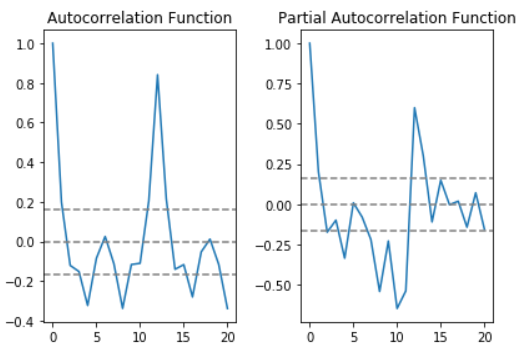• p：部分自相关函数表第一次截断的上层置信区间是滞后值。如果你仔细看，该值是p=0
• q：自相关函数表第一次截断的上层置信区间是滞后值。如果你仔细看，该值是q=1

from statsmodels.tsa.arima_model import ARIMA

model = ARIMA(ts_log, order=(0, 1, 0))
results_AR = model.fit(disp=-1)
plt.plot(ts_log_diff)
plt.plot(results_AR.fittedvalues, color='red')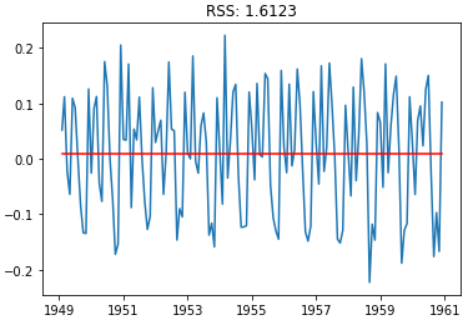model = ARIMA(ts_log, order=(0, 1, 1))
results_MA = model.fit(disp=-1)
plt.plot(ts_log_diff)
plt.plot(results_MA.fittedvalues, color='red')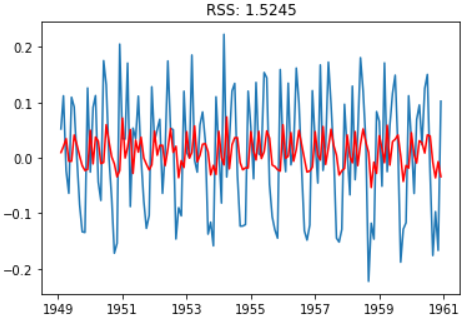ARMA组合

model = ARIMA(ts_log, order=(0, 1, 1))
results_ARIMA = model.fit(disp=-1)
plt.plot(ts_log_diff)
plt.plot(results_ARIMA.fittedvalues, color='red')

predictions_ARIMA_diff = pd.Series(results_ARIMA.fittedvalues, copy=True)
TravelDate
1949-02-01    0.009726
1949-03-01    0.020485
1949-04-01    0.034537
1949-05-01   -0.005924
1949-06-01   -0.006085
dtype: float64

predictions_ARIMA_diff_cumsum = predictions_ARIMA_diff.cumsum()
TravelDate
1949-02-01    0.009726
1949-03-01    0.030211
1949-04-01    0.064748
1949-05-01    0.058824
1949-06-01    0.052739
dtype: float64

predictions_ARIMA_log = pd.Series(ts_log.iloc, index=ts_log.index)
TravelDate
1949-01-01    4.718499
1949-02-01    4.728225
1949-03-01    4.748710
1949-04-01    4.783247
1949-05-01    4.777323
dtype: float64

predictions_ARIMA = np.exp(predictions_ARIMA_log)
plt.plot(ts)
plt.plot(predictions_ARIMA)
plt.title('RMSE: %.4f'% np.sqrt(sum((predictions_ARIMA-ts)**2)/len(ts)))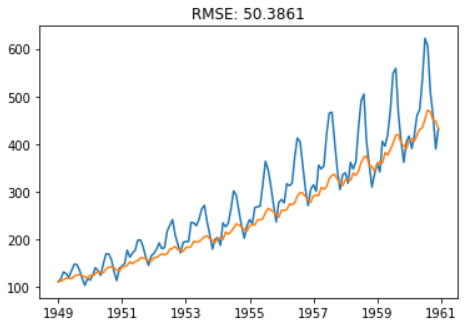ARIMA模型实战，基于“分解”进行未来预测

from statsmodels.tsa.arima_model import ARIMA

residual.dropna(inplace=True)
model_ARIMA = ARIMA(residual,(0,1,1)).fit(disp=-1, method='css')
predictions_ARIMA = model_ARIMA.predict(start='1950-01-01',end='1962-04-01')
plt.plot(residual)
plt.plot(predictions_ARIMA)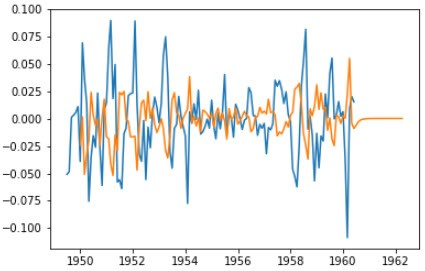predictions_ARIMA = np.exp(predictions_ARIMA)
plt.plot(data['Passengers'],color='blue')
plt.plot(predictions_ARIMA,color='red')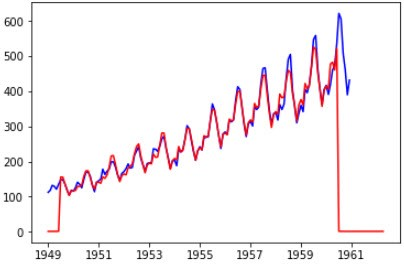print(trend)
print(seasonal)

plt.plot(trend)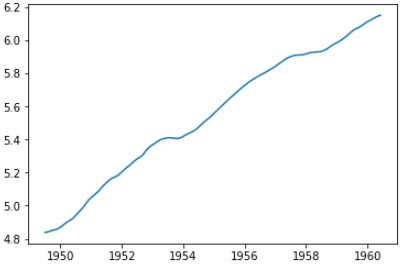trend.dropna(inplace=True)
from sklearn.linear_model import LinearRegression
x = pd.Series(range(trend.size),index=trend.index)
x = x.to_frame()
linreg = LinearRegression()
linereg = linreg.fit(x, trend)
x = pd.Series(range(0,154),index=(pd.period_range('1949-07',periods=154,freq = 'M')))
x = x.to_frame()
res_predict = linereg.predict(x)
trend2 = pd.Series(res_predict,index=x.index).to_timestamp()

plt.plot(trend,color='blue')
plt.plot(trend2,color='red')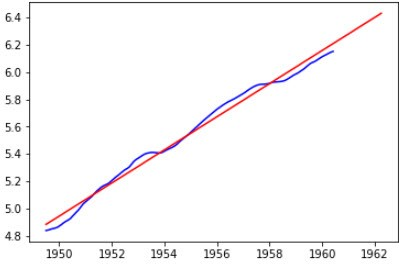index1 = pd.date_range(start='1949-01-01',end='1962-04-01',freq='MS')
seasonal=seasonal.reindex(index1)
seasonal = seasonal.shift(24)
plt.plot(seasonal)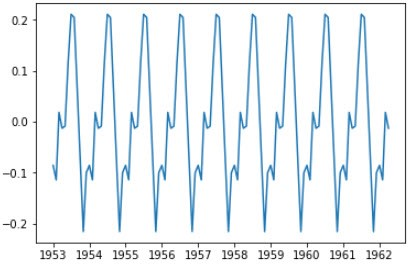model_ARIMA = ARIMA(residual,(0,1,1)).fit(disp=-1, method='css')
predictions_ARIMA = model_ARIMA.predict(start='1950-01-01',end='1962-04-01')
predictions_ARIMA = np.exp(predictions_ARIMA)
plt.plot(data['Passengers'],color='blue')
plt.plot(predictions_ARIMA,color='red')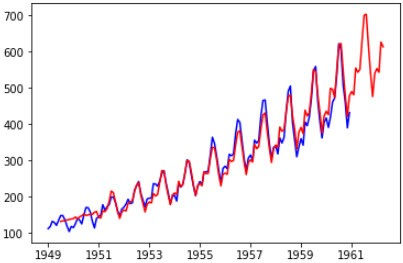ARIMA模型实战，基于网格搜索确定参数

from statsmodels.tsa.arima_model import ARIMA  # 导入ARIMA模型
d = 1 # 一阶差分后平稳，所以d=1。p,q参数使用循环暴力调参确定最佳值
pdq_result = pd.DataFrame()
for p in range(0, 5):
for q in range(0, 5):
try:
model = ARIMA(ts_week_log,order=(p, d, q))  # ARIMA的参数: order = p, d, q
results_ARIMA = model.fit()
pdq_result = pdq_result.append(pd.DataFrame(
{'p': [p], 'd': [d],'q': [q], 'AIC': [results_ARIMA.aic], 'BIC': [results_ARIMA.bic],
'HQIC':[results_ARIMA.hqic]}))
#print(results_ARIMA.summary())  #summary2()也可以使用
except:
pass
print(pdq_result)
p  d  q           AIC           BIC          HQIC
0  0  1  0 -13939.315506 -13927.843765 -13935.132034
0  0  1  1 -14083.366353 -14066.158742 -14077.091145
0  0  1  2 -14083.421767 -14060.478286 -14075.054823
0  0  1  3 -14081.931844 -14053.252492 -14071.473164
0  0  1  4 -14080.862357 -14046.447135 -14068.311941
0  1  1  0 -14083.792730 -14066.585119 -14077.517522
0  1  1  1 -14083.699762 -14060.756281 -14075.332818
0  1  1  2 -14082.602763 -14053.923412 -14072.144084
0  1  1  4 -14078.946543 -14038.795451 -14064.304391
0  2  1  0 -14083.401327 -14060.457845 -14075.034383
0  2  1  1 -14081.986100 -14053.306749 -14071.527420
0  2  1  2 -14081.512486 -14047.097264 -14068.962070
0  3  1  0 -14082.610717 -14053.931365 -14072.152037
0  3  1  1 -14080.785531 -14046.370309 -14068.235115
0  3  1  2 -14087.953053 -14047.801961 -14073.310901
0  3  1  3 -14086.313032 -14040.426069 -14069.579144
0  4  1  0 -14080.937459 -14046.522237 -14068.387043
0  4  1  1 -14078.958559 -14038.807467 -14064.316408
0  4  1  2 -14086.257204 -14040.370241 -14069.523316
0  4  1  4 -14082.963600 -14025.604897 -14062.046240

model =ARIMA(ts_week_log, order=(3, 1, 2))  # ARIMA的参数: order = p, d, q
results_ARIMA =model.fit()
plt.plot(ts_week_log_diff)
plt.plot(results_ARIMA.fittedvalues, color='red')  # fittedvalues返回的是d次差分后的序列, 因此和ts_week_log_diff比较
residuals=pd.DataFrame(results_ARIMA.resid) # 拟合的数据和原始数据ts_week_log的残差
plt.show()

acf_pacf(residuals,lags=10,title='Residuals') #等于acf_pacf(results_ARIMA.fittedvalues-ts_week_log_diff,lags=10,title='Residuals')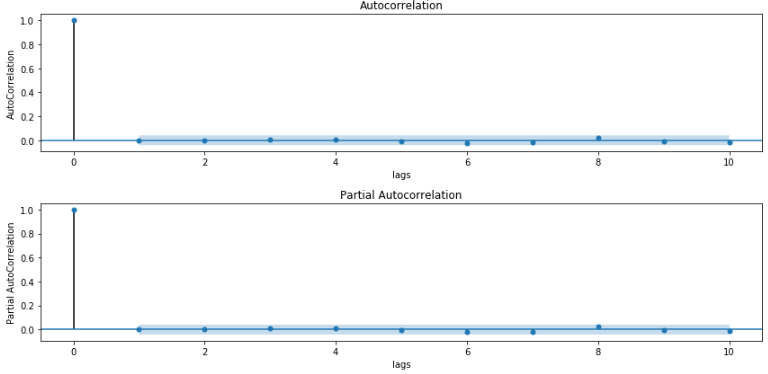print('Residuals Summary:\n', residuals.describe())
residuals.plot(kind='kde') #概率密度图kde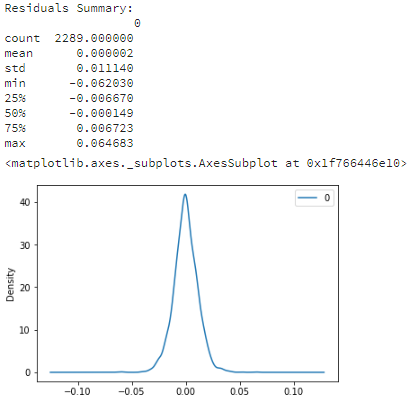predictions_ARIMA_diff= pd.Series(results_ARIMA.fittedvalues, copy=True)  # 复制Log和差分后的预测值
predictions_ARIMA_diff_cumsum= predictions_ARIMA_diff.cumsum()  # 一阶差分的逆向转换为累计和
predictions_ARIMA_log= pd.Series(ts_week_log.iloc, index=ts_week_log.index)  #将一个新序列的每个元素都填充为ts_week_log的第一个元素
predictions_ARIMA= np.exp(predictions_ARIMA_log)  # 进行指数运算，还原为原始值
plt.plot(ts_week)
plt.plot(predictions_ARIMA)
plt.title('RMSE:%.4f' % np.sqrt(sum((predictions_ARIMA - ts_week) ** 2) / len(ts_week)))
plt.show()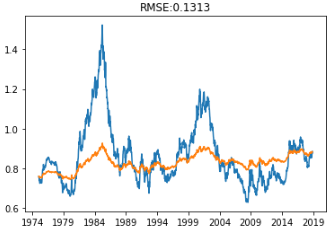from sklearn.metrics import mean_squared_error
from statsmodels.tsa.arima_model import ARIMA

pdq = (3, 1, 2)
test_point = 15 # 测试点数
size = len(ts_week_log) - 15
train, test = ts_week_log[0:size], ts_week_log[size:len(ts_week_log)] #切分数据集

model = ARIMA(train, order=pdq)
model_fit = model.fit()
predictions = model_fit.predict(start=size,end=size+test_point-1, dynamic=True).tolist()

error = mean_squared_error(test, predictions)
print('Printing Mean Squared Error of Predictions...')

predictions_series = pd.Series(predictions, index = test.index) # predictions没有Index，无法在图上显示出来，必须先追加Index

fig, ax = plt.subplots()
ax.set(title='Spot Exchange Rate, Euro into USD', xlabel='Date', ylabel='Euro into USD')
ax.plot(ts_week[-50:], 'o', label='observed')
ax.plot(np.exp(euro_predictions_series), 'g', label='rolling one-step out-of-sample forecast')
legend = ax.legend(loc='upper left')
legend.get_frame().set_facecolor('w')

SARIMAX

SARIMAX模型简介

ARIMA是用于单变量时间序列数据预测的最广泛使用的预测方法之一。尽管该方法可以处理具有趋势的数据，但它不支持具有季节性组件的时间序列。支持对该系列季节性组成部分进行直接建模的ARIMA扩展称为(SARIMA(Seasonal Auto Regressive Integrated Moving Averages with eXogenous regressors.)。它增加了三个新的参数来指定系列季节性成分的自回归（AR），差分（I）和移动平均（MA），以及季节性周期的附加参数。

ARIMA -> SARIMA -> SARIMAX：

• S是Seasonal，就是季节性、周期性的意思
• X是eXogenous，外部信息的意思

SARIMAX模型的使用

SARIMAX模型与ARIMA最大的不同是，会多出季节性参数。需要提供的参数为：

• p：趋势自回归阶数
• d：趋势差分阶数
• q：趋势移动平均阶数

• P：季节性自回归阶数
• D：季节性差分阶数
• Q：季节性移动平均阶数
• m：单个季节期间的时间步数

• 假如序列有显著是季节性模式，则需要用季节性差分，但是不要使用两次季节性差分或总过超过两次差分（季节性和非季节性）
• 假如差分序列在滞后s处有正的ACF，s是季节的长度，则需要加入SAR项；假如差分序列在滞后s处有负的ACF，则需要加入SMA项，如果使用了季节性差异，则后一种情况很可能发生，如果数据具有稳定和合乎逻辑的季节性模式。如果没有使用季节性差异，前者很可能会发生，只有当季节性模式不稳定时才适用。应该尽量避免在同一模型中使用多于一个或两个季节性参数（SAR + SMA），因为这可能导致过度拟合数据和/或估算中的问题。

SARIMAX模型实战

import itertools
import pandas as pd
import numpy as np
import statsmodels.api as sm
import matplotlib.pyplot as plt
plt.style.use('fivethirtyeight')

data = data['co2'].resample('MS').mean()
data = data.fillna(data.bfill())
data.plot(figsize=(12, 6))
plt.show()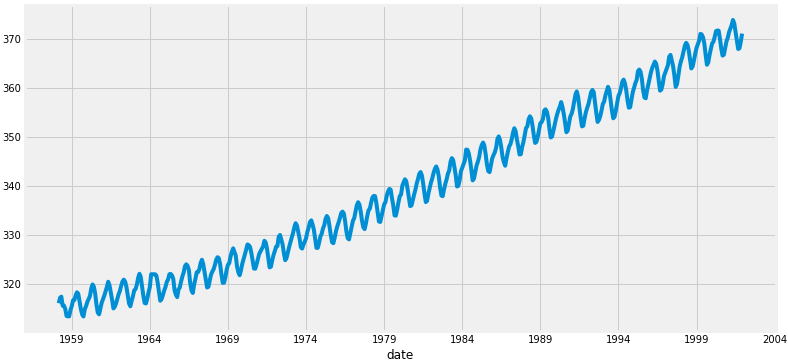# Define the p, d and q parameters to take any value between 0 and 2
p = d = q = range(0, 2)

# Generate all different combinations of p, q and q triplets
pdq = list(itertools.product(p, d, q))
print(pdq)

# Generate all different combinations of seasonal p, q and q triplets
seasonal_pdq = [(x, x, x, 12) for x in list(itertools.product(p, d, q))]
print(seasonal_pdq)

import warnings
warnings.filterwarnings("ignore") # specify to ignore warning messages

for param in pdq:
for param_seasonal in seasonal_pdq:
try:
model = sm.tsa.statespace.SARIMAX(data, order=param, seasonal_order=param_seasonal, enforce_stationarity=False, enforce_invertibility=False)
results = model.fit()
print('ARIMA{}x{} - AIC:{}'.format(param, param_seasonal, results.aic))
except:
continue

ARIMA(0, 0, 0)x(0, 0, 0, 12) - AIC:7612.583429881011
ARIMA(0, 0, 0)x(0, 0, 1, 12) - AIC:6787.343624031752
ARIMA(0, 0, 0)x(0, 1, 0, 12) - AIC:1854.828234141261
ARIMA(0, 0, 0)x(0, 1, 1, 12) - AIC:1596.711172763898
ARIMA(0, 0, 0)x(1, 0, 0, 12) - AIC:1058.9388921320026
ARIMA(0, 0, 0)x(1, 0, 1, 12) - AIC:1056.2878557033246
ARIMA(0, 0, 0)x(1, 1, 0, 12) - AIC:1361.6578978072075
ARIMA(0, 0, 0)x(1, 1, 1, 12) - AIC:1044.7647912998186
ARIMA(0, 0, 1)x(0, 0, 0, 12) - AIC:6881.048755126321
ARIMA(0, 0, 1)x(0, 0, 1, 12) - AIC:6072.662327947137
ARIMA(0, 0, 1)x(0, 1, 0, 12) - AIC:1379.1941067330986
ARIMA(0, 0, 1)x(0, 1, 1, 12) - AIC:1241.4174716851521
ARIMA(0, 0, 1)x(1, 0, 0, 12) - AIC:1084.9453872064087
ARIMA(0, 0, 1)x(1, 0, 1, 12) - AIC:780.4316223926196
ARIMA(0, 0, 1)x(1, 1, 0, 12) - AIC:1119.5957893630987
ARIMA(0, 0, 1)x(1, 1, 1, 12) - AIC:807.0912988896297
ARIMA(0, 1, 0)x(0, 0, 0, 12) - AIC:1675.8086923024293
ARIMA(0, 1, 0)x(0, 0, 1, 12) - AIC:1240.2211199194085
ARIMA(0, 1, 0)x(0, 1, 0, 12) - AIC:633.4425586468699
ARIMA(0, 1, 0)x(0, 1, 1, 12) - AIC:337.793854858494
ARIMA(0, 1, 0)x(1, 0, 0, 12) - AIC:619.9501759055394
ARIMA(0, 1, 0)x(1, 0, 1, 12) - AIC:376.9283760898942
ARIMA(0, 1, 0)x(1, 1, 0, 12) - AIC:478.3296906672489
ARIMA(0, 1, 0)x(1, 1, 1, 12) - AIC:323.07764996953097
ARIMA(0, 1, 1)x(0, 0, 0, 12) - AIC:1371.187260233786
ARIMA(0, 1, 1)x(0, 0, 1, 12) - AIC:1101.8410734303056
ARIMA(0, 1, 1)x(0, 1, 0, 12) - AIC:587.9479709744935
ARIMA(0, 1, 1)x(0, 1, 1, 12) - AIC:302.49490008406354
ARIMA(0, 1, 1)x(1, 0, 0, 12) - AIC:584.4333533493711
ARIMA(0, 1, 1)x(1, 0, 1, 12) - AIC:337.1999050779197
ARIMA(0, 1, 1)x(1, 1, 0, 12) - AIC:433.0863608210692
ARIMA(0, 1, 1)x(1, 1, 1, 12) - AIC:281.5190053393452
ARIMA(1, 0, 0)x(0, 0, 0, 12) - AIC:1676.8881767362054
ARIMA(1, 0, 0)x(0, 0, 1, 12) - AIC:1241.9353188506311
ARIMA(1, 0, 0)x(0, 1, 0, 12) - AIC:624.2602350563734
ARIMA(1, 0, 0)x(0, 1, 1, 12) - AIC:341.2896611730041
ARIMA(1, 0, 0)x(1, 0, 0, 12) - AIC:579.3896901160358
ARIMA(1, 0, 0)x(1, 0, 1, 12) - AIC:370.591911050293
ARIMA(1, 0, 0)x(1, 1, 0, 12) - AIC:476.0500429124218
ARIMA(1, 0, 0)x(1, 1, 1, 12) - AIC:329.5844988945166
ARIMA(1, 0, 1)x(0, 0, 0, 12) - AIC:1372.6085881684037
ARIMA(1, 0, 1)x(0, 0, 1, 12) - AIC:1199.4888202249872
ARIMA(1, 0, 1)x(0, 1, 0, 12) - AIC:586.4485732044088
ARIMA(1, 0, 1)x(0, 1, 1, 12) - AIC:305.62738298712236
ARIMA(1, 0, 1)x(1, 0, 0, 12) - AIC:586.5467067203238
ARIMA(1, 0, 1)x(1, 0, 1, 12) - AIC:400.3606781886424
ARIMA(1, 0, 1)x(1, 1, 0, 12) - AIC:433.54694646294087
ARIMA(1, 0, 1)x(1, 1, 1, 12) - AIC:284.35964168784733
ARIMA(1, 1, 0)x(0, 0, 0, 12) - AIC:1324.311112732457
ARIMA(1, 1, 0)x(0, 0, 1, 12) - AIC:1060.935191442204
ARIMA(1, 1, 0)x(0, 1, 0, 12) - AIC:600.7412682874252
ARIMA(1, 1, 0)x(0, 1, 1, 12) - AIC:312.1329632683155
ARIMA(1, 1, 0)x(1, 0, 0, 12) - AIC:593.6637754853627
ARIMA(1, 1, 0)x(1, 0, 1, 12) - AIC:349.209146507186
ARIMA(1, 1, 0)x(1, 1, 0, 12) - AIC:440.1375884358338
ARIMA(1, 1, 0)x(1, 1, 1, 12) - AIC:293.56145595783397
ARIMA(1, 1, 1)x(0, 0, 0, 12) - AIC:1262.6545542448305
ARIMA(1, 1, 1)x(0, 0, 1, 12) - AIC:1052.0636724058634
ARIMA(1, 1, 1)x(0, 1, 0, 12) - AIC:581.3099935252751
ARIMA(1, 1, 1)x(0, 1, 1, 12) - AIC:295.9374058139739
ARIMA(1, 1, 1)x(1, 0, 0, 12) - AIC:576.8647112079228
ARIMA(1, 1, 1)x(1, 0, 1, 12) - AIC:327.90491219439946
ARIMA(1, 1, 1)x(1, 1, 0, 12) - AIC:444.12436865154666
ARIMA(1, 1, 1)x(1, 1, 1, 12) - AIC:277.7802201071108

model = sm.tsa.statespace.SARIMAX(data, order=(1, 1, 1), seasonal_order=(1, 1, 1, 12), enforce_stationarity=False, enforce_invertibility=False)
results = model.fit()
print(results.summary().tables)

==============================================================================
coef    std err          z      P>|z|      [0.025      0.975]
------------------------------------------------------------------------------
ar.L1          0.3182      0.092      3.442      0.001       0.137       0.499
ma.L1         -0.6254      0.077     -8.163      0.000      -0.776      -0.475
ar.S.L12       0.0010      0.001      1.732      0.083      -0.000       0.002
ma.S.L12      -0.8769      0.026    -33.812      0.000      -0.928      -0.826
sigma2         0.0972      0.004     22.632      0.000       0.089       0.106
==============================================================================

results.plot_diagnostics(figsize=(12, 12))
plt.show()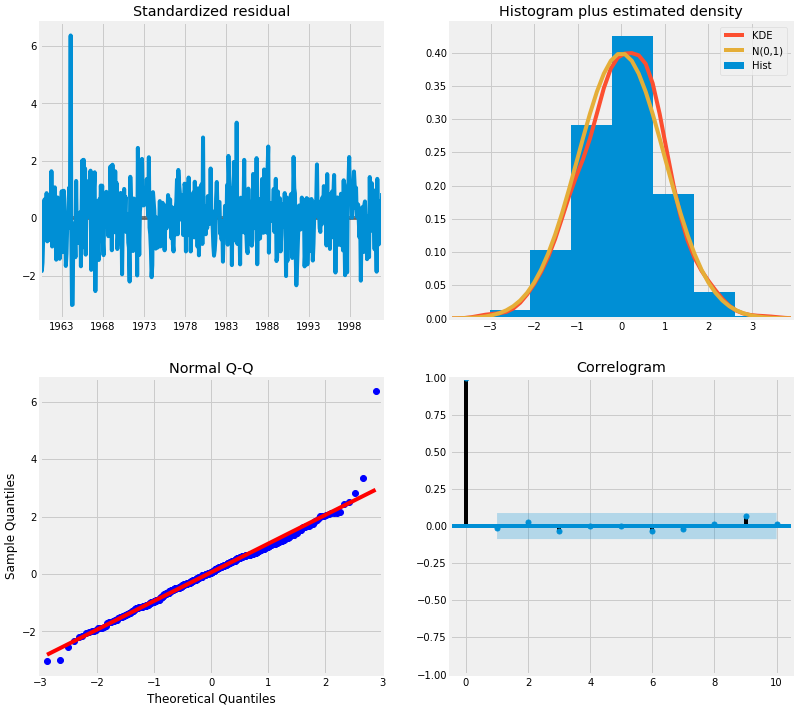• 右上角的红色KDE线和黄色N(0,1)线非常的接近（N(0,1)表示，平均值为0，标准差为1），表明残差是正态分布。
• 左下角对的QQ分位图表明，从N(0,1)的标准正态分布抽取的样本，残差(蓝点)的有序分布服从线性趋势。 同样，这是一个强烈的迹象表明，残差是正态分布。
• 随着时间的推移（左上图）残差不会显示任何明显的季节性，似乎是白噪声。这通过右下角的自相关（即相关图）证实了这一点，它表明时间序列的残差与其自身的滞后版本有很低的相关性。

pred = results.get_prediction(start=pd.to_datetime('1998-01-01'), dynamic=False)
pred_ci = pred.conf_int()

ax = data['1990':].plot(label='Observed',figsize=(12, 6))

ax.fill_between(pred_ci.index,
pred_ci.iloc[:, 0],
pred_ci.iloc[:, 1], color='k', alpha=.2)

ax.set_xlabel('Date')
ax.set_ylabel('CO2 Levels')
plt.legend()

plt.show()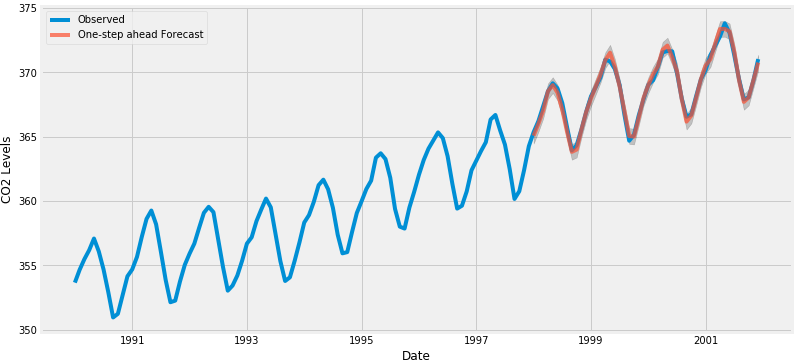data_forecasted = pred.predicted_mean
data_truth = data['1998-01-01':]

# Compute the mean square error
mse = ((data_forecasted - data_truth) ** 2).mean()
print('The Mean Squared Error of our forecasts is {}'.format(round(mse, 2)))

pred_dynamic = results.get_prediction(start=pd.to_datetime('1998-01-01'), dynamic=True, full_results=True)
pred_dynamic_ci = pred_dynamic.conf_int()

ax = data['1990':].plot(label='Observed', figsize=(12, 6))
pred_dynamic.predicted_mean.plot(label='Dynamic Forecast', ax=ax)

ax.fill_between(pred_dynamic_ci.index,
pred_dynamic_ci.iloc[:, 0],
pred_dynamic_ci.iloc[:, 1], color='k', alpha=.25)

ax.fill_betweenx(ax.get_ylim(), pd.to_datetime('1998-01-01'), data.index[-1],
alpha=.1, zorder=-1)

ax.set_xlabel('Date')
ax.set_ylabel('CO2 Levels')

plt.legend()
plt.show()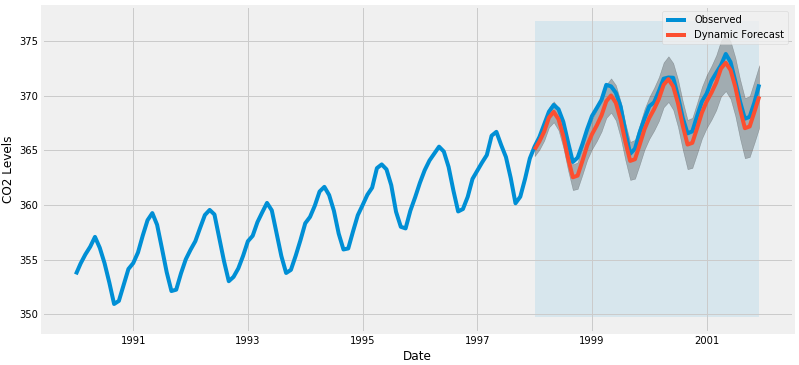# Extract the predicted and true values of our time series
data_forecasted = pred_dynamic.predicted_mean
data_truth = data['1998-01-01':]

# Compute the mean square error
mse = ((data_forecasted - data_truth) ** 2).mean()
print('The Mean Squared Error of our forecasts is {}'.format(round(mse, 2)))

# Get forecast 500 steps ahead in future
pred_uc = results.get_forecast(steps=500)

# Get confidence intervals of forecasts
pred_ci = pred_uc.conf_int()

ax = data.plot(label='Observed', figsize=(12, 6))
pred_uc.predicted_mean.plot(ax=ax, label='Forecast')
ax.fill_between(pred_ci.index,
pred_ci.iloc[:, 0],
pred_ci.iloc[:, 1], color='k', alpha=.25)
ax.set_xlabel('Date')
ax.set_ylabel('CO2 Levels')

plt.legend()
plt.show()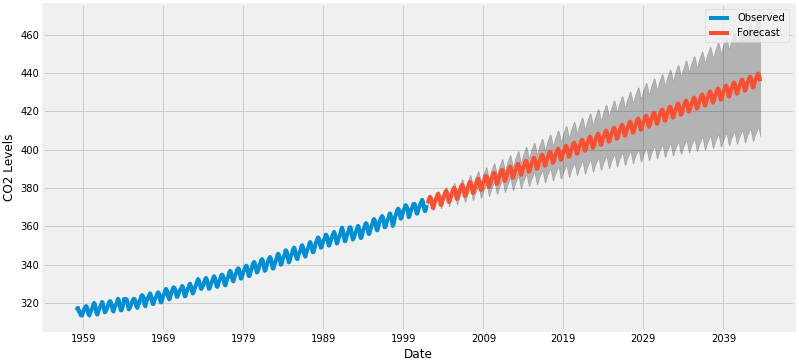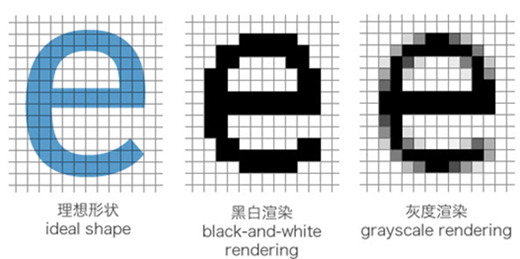网站与APP开发中的字体设置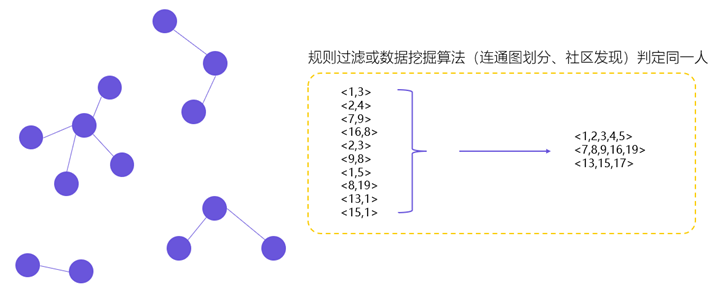用户体系搭建之ID-Mapping